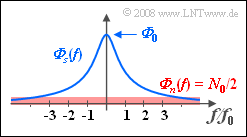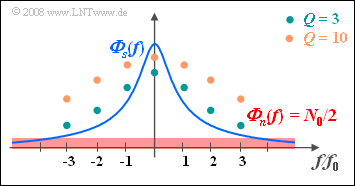# Exercise 5.9: Minimization of the MSEPower-spectral densities
with the Wiener filter

Given a stochastic signal  $s(t)$ of which only the power-spectral density  $\rm (PSD)$  is known:

$${\it \Phi} _s (f) = \frac{\it{\Phi} _{\rm 0} }{1 + ( {f/f_0 } )^2 }.$$

This power-spectral density  ${\it \Phi} _s (f)$  is shown in blue in the accompanying diagram.

• The average power of  $s(t)$  is obtained by integration over the power-spectral density:
$$P_s = \int_{ - \infty }^{ + \infty } {{\it \Phi} _s (f)}\, {\rm d} f = {\it \Phi} _0 \cdot f_0 \cdot {\rm{\pi }}.$$
• Additively superimposed on this signal  $s(t)$  is white noise  $n(t)$  with noise power density  ${\it \Phi}_n(f) = N_0/2.$
• As an abbreviation,  we use  $Q = 2 \cdot {\it \Phi}_0/N_0$,  where  $Q$  could be interpreted as  "quality".
• Note that  $Q$  does not represent a signal–to–noise power ratio.

In this exercise,  we want to determine the frequency response  $H(f)$  of a filter that minimizes the mean square error  $\rm (MSE)$  between the useful signal  $s(t)$  and the filter output signal  $d(t)$:

$${\rm{MSE}} = \mathop {\lim }\limits_{T_{\rm M} \to \infty } \frac{1}{T_{\rm M} }\int_{ - T_{\rm M} /2}^{T_{\rm M} /2} {\left| {d(t) - s(t)} \right|^2 \, {\rm{d}}t.}$$

Notes:

• The exercise belongs to the chapter  Wiener–Kolmogorow Filter.
• For the optimal frequency response,  according to Wiener and Kolmogorov,  the following applies in general:
$$H_{\rm WF} (f) = \frac{1}{{1 + {\it \Phi} _n (f)/{\it \Phi} _s (f)}}.$$
• The following indefinite integral is given for solving:
$$\int {\frac{1}{a^2 + x^2 }} \, {\rm{d}}x ={1}/{a} \cdot \arctan \left( {{x}/{a}} \right).$$

### Questions

1

Which of the following statements are true?

 $H(f)$  is a Gaussian low-pass. $H(f)$  represents a matched filter. $H(f)$  represents a Wiener–Kolmogorov filter.

2

Determine the frequency response  $H(f)=H_{\rm WF} (f)$  of the optimum filter for this purpose.
What values result with  $Q = 3$  at  $f = 0$  and  $f = 2f_0$?

 $H(f = 0) \ = \$ $H(f = 2f_0)\ = \$

3

Let  $Q = 3$.  Calculate the mean square error  $(\rm MSE)$  with respect to  $P_s$  of the best possible filter.

 ${\rm MSE}/P_s \ = \$

4

How must the  "quality factor"  $Q$  be chosen at least,  so that for the quotient the value  ${\rm MSE}/P_s = 0.1$  can be reached?

 $Q_\text{min} \ = \$

5

Which of the following statements are true?

 A filter of the same shape  $H(f) = K \cdot H_{\rm WF}(f)$  leads to the same result. The output signal  $d(t)$  contains more higher frequency components when  $Q$  is larger.

### Solution

#### Solution

(1)  Only the last solution  is correct:

• The task  "Minimization of the mean square error"  already points to the Wiener–Kolmogorow filter.
• The matched filter,  on the other hand,  is used to concentrate the signal energy and thereby maximize the S/N ratio for a given time.

(2)  For the optimal frequency response,  according to Wiener and Kolmogorov,  the following applies in general:

$$H(f) = H_{\rm WF} (f) = \frac{1}{{1 + {\it \Phi} _n (f)/{\it \Phi} _s (f)}}.$$
• With the given power-spectral density,  it is also possible to write for this:
$$H(f) = \frac{1}{{1 + {N_0 }/({{2{\it \Phi} _0 })}\cdot \left[ {1 + ( {f/f_0 } )^2 } \right]}} = \frac{1}{{1 + {1}/{Q}\cdot \left[ {1 + ( {f/f_0 } )^2 } \right]}}.$$
• With  $Q = 3$  it follows:
$$H( {f = 0} ) = \frac{1}{{1 + {1}/{Q}}} = \frac{Q}{Q + 1} \hspace{0.15cm}\underline {= 0.75},$$
$$H( {f = 2f_0 } ) = \frac{1}{{1 + {5}/{Q}}} = \frac{Q}{Q + 5} \hspace{0.15cm}\underline {= 0.375}.$$

(3)  For the filter calculated in subtask  (2),  taking symmetry into account,  the following holds:

$${\rm{MSE = }}\int_{-\infty}^{+\infty} H(f) \cdot {\it \Phi} _n (f) \,\, {\rm{d}}f = \int_{0}^{+\infty} \frac{N_0}{{1 + {N_0 }/({{2{\it \Phi} _0 })}\cdot \left[ {1 + ( {f/f_0 } )^2 } \right]}} \,\, {\rm{d}}f .$$
• For this,  with  $Q = 2 \cdot {\it \Phi}_0/N_0$  and  $a^2 = Q + 1$,  we can also write:
$${\rm{MSE = }}\int_0^\infty {\frac{{2{\it \Phi} _0 }}{{ Q+1 + ( {f/f_0 })^2 }}} \,\, {\rm{d}}f = 2{\it \Phi} _0 \cdot f_0 \int_0^\infty {\frac{1}{a^2 + x^2 }}\,\, {\rm{d}}x.$$
• This leads to the result with the integral given:
$${\rm{MSE}} = \frac{{2{\it \Phi} _0 f_0 }}{{\sqrt {1 + Q} }}\left( {\arctan ( \infty ) - \arctan ( 0 )} \right) = \frac{{{\it \Phi} _0 f_0 {\rm{\pi }}}}{{\sqrt {1 + Q} }}.$$
• Normalizing MSE to the power  $P_s$  of the  signal $s(t)$,  we obtain for  $Q=3$:
$$\frac{\rm{MSE}}{P_s} = \frac{1}{{\sqrt {1 + Q} }} \hspace{0.15cm}\underline { = 0.5}.$$

(4)  From the calculation in subtask  (3),  the condition  $Q \ge 99$   ⇒   $Q_{\rm min} \hspace{0.15cm}\underline{= 99}$ follows directly for  ${\rm MSE}/P_s \ge 0.1.$

• The larger  $Q$,  the smaller the mean square error becomes.

(5)  Only the second solution  is correct:

• A frequency response of the same shape as the Wiener–Kolmogorov filter   ⇒   $H(f) = K \cdot H_{\rm WF}(f)$  with  $K \ne 1$  always leads to large distortions.
• This can be illustrated by the noise-free case  $(Q \to \infty)$:  $d(t) = K \cdot s(t)$  and the optimization task would be extremely poorly solved despite good conditions.
• One might incorrectly conclude from the equationPower-spectral density with the
Wiener–Kolmogorov filter
$${\rm{MSE}} = \int_{ - \infty }^{ + \infty } {H_{\rm WF} (f)} \cdot \it{\Phi} _n (f)\,\,{\rm{d}}f$$
that a filter  $H(f) = 2 \cdot H_{\rm WF}(f)$  only doubles the mean squared error.
• However,  this is not the case,  since  $H(f)$  is then no longer a Wiener filter and the above equation is no longer applicable.

The second statement is true,  as can be seen from the accompanying diagram.

• The dots mark the frequency response  $H_{\rm WF}(f)$  of the filter for  $Q = 3$  and for  $Q = 10$, resp.
• For larger  $Q (= 10)$,  high components are attenuated less than for lower  $Q (= 3)$.
• Therefore,  in the case of  $Q = 10$,  the filter output signal contains more higher frequency components,  which are due to the noise  $n(t)$.